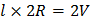# Consider a rectangular slab of lengthand area of cross sectionA currentis passed through it. If the length is doubled, the potential drop across the end faces a) Becomes half of the initial value b) Becomes one-fourth of the initial value c) Becomes double the initial value d) Remains same

## Question ID - 150218 :- Consider a rectangular slab of lengthand area of cross sectionA currentis passed through it. If the length is doubled, the potential drop across the end faces a) Becomes half of the initial value b) Becomes one-fourth of the initial value c) Becomes double the initial value d) Remains same

3537

(c)

Resistance of the slabwhereThe potential acrossisThe length of the slab is doubled. Therefore the resistance isAssuming that the same current is passed, the potential across the new resistance isNext Question :

Two cells having emf 4V, 2V and internal resistances 11are connected as shown in figure below. Current through 6resistance isa)b)c) 1A d)